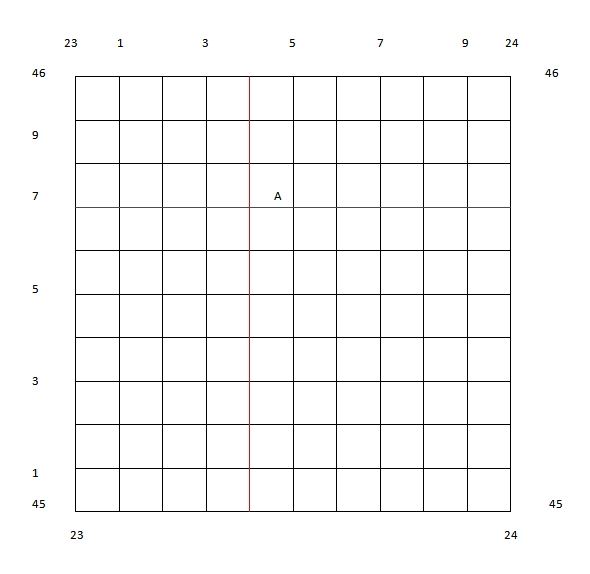Select Page

# 6 Figure Grid References

Four figure grid references are indeed very useful. However, a major weakness of four figure grid references is the fact that they are not very accurate. All objects in the same grid square have the same four figure grid reference even though they may be hundreds of meters apart . When greater accuracy is necessary, a six figure grid reference is used.A six figure grid reference does not only indicate the grid square an object is located in. It also tells us the exact point within the grid square where the object is found. Therefore, objects located in the same grid square will have the same four figure grid reference, but different six figure grid references.

## How to Give a Six Figure Grid Reference

A six figure grid reference takes the form EEXNNY. EE represents the easting which is immediately to the left of the object and NN represents the northing which is directly under it. Therefore EE and NN represent the four figure grid reference for the object in question. X is a digit which tells us how close to or far away from the easting the object is located. The higher the number, the farther away from the easting the object is. Similarly, Y is a digit which tells us how close to or far away from the northing the object is found. X and Y can have a value ranging from zero to nine. Look at the grid square below. Look at the letter “A” located within the square. The four figure grid reference for “A” is 2345. However, 2345 is also the four figure grid reference for any object which lies anywhere in this grid square. We can give the exact location of this “A” by giving a six figure grid reference. Let us take it step by step.### Step 1

Remember a six figure grid reference takes the form EEXNNY. The first two digits represent the easting immediately to the left of the object. The easting to the left of “A” is 23, therefore we have our first two digits. The third digit (X) represents the distance between easting 23 and “A”. To determine this we need to divide the space between easting 23 and easting 24 into ten equal parts as seen in the diagram below.The lines are parallel to our easting are an equal distance apart from each other. Let’s call these lines “mini eastings”. Now we need to count the number of mini eastings that are between easting 23 and “A”. In this case there are four. Therefore our third digit is 4. So the first part of our six figure grid reference is 234.

### Step 2

The fourth and fifth digits in a six figure grid reference represent the northing which is directly under the object. In this case it is northing 45. The sixth digit tells us the distance between northing 45 and the object. We must divide the space between northings 45 and 46 into ten equal parts as seen below.Once again the lines are parallel to northing 45 and are an equal distance apart. Let us call these lines “mini northings”. We must count the number of mini northings which are between northing 45 and “A”. In this case there are seven of them. Therefore 7 will be the last digit in our six figure grid reference. The second part of our grid reference is 457.

Our entire six figure grid reference is 234457. Remember:

• the first two digits represent the easting immediately to the left of the object( in this case easting 23).
• the third digit (4) represents the distance between the easting and our object. In this case the object is roughly four tenths of the distance between easting 23 and easting 24.
• the fourth and fifth digits represent the northing directly under our object (in this case northing 45)
• the sixth digit represents the distance between the northing and our object. In this case, the object is roughly seven tenths of the distance between northing 45 and northing 46.

Note: If the position of “A” was such that it lay directly on mini easting 4 or mini northing 7, its six figure grid reference would still be 234457.

The two steps shown above need not be done separately. Your “mini eastings” and “mini northings” can be drawn such that they form a smaller grid inside the grid square in which the object is located. The six figure grid reference can be completed by giving the number of the mini easting immediately to the left of the object and the number of the mini easting directly under it (see below).The six figure grid reference is 234457

Take the Six Figure Grid Reference Quiz!